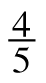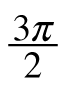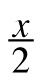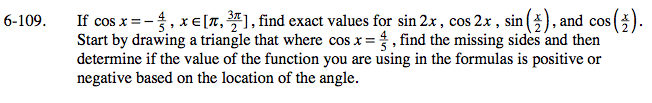### Home > PC > Chapter 6 > Lesson 6.3.1 > Problem6-109

6-109.

If cos x = −, x [π,], find exact values for sin 2x, cos 2x, sin (), and cos (). Start by drawing a triangle that where cos x =, find the missing sides and then determine if the value of the function you are using in the formulas is positive or negative based on the location of the angle. Homework Help ✎Double - Angle Formulas
sin(2α) = 2sin(α)cos(α)
cos(2α) = cos2(α) − sin2(α)

Half - Angle Formulas

$\sin\left ( \frac{\theta }{2} \right )=\pm \sqrt{\frac{1-\cos(\theta)}{2}}$

$\cos\left(\frac{\theta }{2} \right )=\pm \sqrt{\frac{1+\cos(\theta)}{2}}$

Draw a unit circle and include a right triangle matching the given conditions.
Use the Pythagorean Theorem to determine the value of the missing leg.